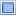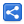# Java - Math.abs() Examples

## Methods:

```public static int abs(int a)
```

Returns the absolute value of an `int` value.

Note that if the argument is equal to the value of Integer.MIN_VALUE, the most negative representable `int` value, the result is that same value, which is negative.

```public static long abs(long a)
```

Returns the absolute value of a `long` value.

Note that if the argument is equal to the value of Long.MIN_VALUE, the most negative representable `long` value, the result is that same value, which is negative.

```public static float abs(float a)
```

Returns the absolute value of a `float` value.

```public static double abs(double a)
```

Returns the absolute value of a `double` value.

## Examples`package com.logicbig.example.math;public class AbsExample {    public static void main(String... args) {        double d = -1.5;        System.out.println("Before: " + d);        double result = Math.abs(d);        System.out.println("After: " + result);    }} `

#### Output

`Before: -1.5After: 1.5``package com.logicbig.example.math;public class AbsExample2 {    public static void main(String... args) {        float f;        f = -1.5f;        System.out.println("Before: " + f);        float result = Math.abs(f);        System.out.println("After: " + result);    }} `

#### Output

`Before: -1.5After: 1.5``package com.logicbig.example.math;public class AbsExample3 {    public static void main(String... args) {        int e = -5;        System.out.println("Before: " + e);        int result = Math.abs(e);        System.out.println("After: " + result);    }} `

#### Output

`Before: -5After: 5``package com.logicbig.example.math;public class AbsExample4 {    public static void main(String... args) {        long g = -543521;        System.out.println("Before: " + g);        long result = Math.abs(g);        System.out.println("After: " + result);    }} `

#### Output

`Before: -543521After: 543521`

abs() can be useful when we want to find the positive difference of two values.`package com.logicbig.example.math;public class AbsExample5 {    public static void main(String... args) {        showDifference(-3, 7);        System.out.println("--------------");        showDifference(-7, -3);        System.out.println("--------------");        showDifference(3, 7);    }    public static void showDifference(int a, int b) {        int diff = a - b;        System.out.printf("Without abs, Diff of %s and %s is %s%n", a, b, diff);        diff = Math.abs(diff);        System.out.printf("With abs, Diff of %s and %s is %s%n", a, b, diff);    }} `

#### Output

`Without abs, Diff of -3 and 7 is -10With abs, Diff of -3 and 7 is 10--------------Without abs, Diff of -7 and -3 is -4With abs, Diff of -7 and -3 is 4--------------Without abs, Diff of 3 and 7 is -4With abs, Diff of 3 and 7 is 4`

 Share